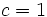# Nilpotent multiplier

Jump to: navigation, search

## Definition

Suppose$c$ is a positive integer. The$c$-nilpotent multiplier of a group$G$, denoted$M^{(c)}(G)$ is defined as the Baer invariant of$G$ with respect to the variety of groups of nilpotency class (at most)$c$. If we write$G = F/R$ where$F$ is a free group, this can be written as:$M^{(c)}(G) = \frac{R \cap \gamma_{c+1}(F)}{[[\dots[R,F],\dots,F],F]}$

where$\gamma_{c+1}(F)$ denotes the$(c+1)^{th}$ member of the lower central series of$F$ and the denominator group has$c$ occurrences of$F$.

## Particular cases

In the case$c = 1$, we get the Schur multiplier.

### Particular cases based on group property

Group property Description of nilpotent multipliers
abelian group nilpotent multiplier of abelian group is graded component of free Lie ring
perfect group nilpotent multiplier of perfect group equals Schur multiplier